Comparison of Intelligent Methods for Thermal Assessment of Power Cables Under Geometrical Parameter Variations | OMICS International
Global Journal of Technology and Optimization

# Comparison of Intelligent Methods for Thermal Assessment of Power Cables Under Geometrical Parameter Variations

M. S. Al-Saud*

Department of Electrical Engineering, King Saud University, Saudi Arabia

*Corresponding Author:
M. S. Al-Saud
Department of Electrical Engineering
King Saud University, Saudi Arabia
E-mail: [email protected]

Received date: January 2012, Revised date: February, Accepted date: April 2012

Visit for more related articles at Global Journal of Technology and Optimization

#### Abstract

In this paper, the thermal field of underground power cable is solved using two intelligent techniques which are newly introduced for thermal assessment of power cables. A backpropagation neural network (BPNN) and an adaptive neuro-fuzzy inference system (ANFIS) models were developed to predict the cable temperature under geometrical parameter variations. The effect of cable spacing and cable burial depth on cable ampacity is investigated. The two models are trained using an input-output pattern generated using finite element (FE) method, which is extensively used in this field. Furthermore, a portion of the FEgenerated output results, which have not been provided to the models – as input data - in the training phase, were utilized to compare the cable temperature of the three methods (FE, ANN and ANFIS). The results of the two intelligent methods show high agreement with the finite element solution, which confirms that introducing intelligent techniques provides a reliable and simple alternative approach for the thermal field evaluation by avoiding the numerous computational complexities of the numerical methods

#### Keywords

Underground cables, ampacity, thermal, finite element methods, neural network, Adaptive neurofuzzy inference system.

#### Introduction

The use of underground power distribution has grown significantly over the years with the rapid increase in demand for electric energy and the trend for large infra-structures and vast expansion of highly-populated metropolitan areas. It is of paramount importance to have an accurate assessment of the maximum allowable current which a cable can tolerate through its life without risking deterioration or damage. The actual current rating of an electric cable is based on the thermal environment in which the cable is installed. The traditional method of computing temperature distribution and cable ampacity is based on approximate formulas derived first by  in 1957 and adopted later by the International Electro-technical Commission (IEC). In particular, the ambient temperature, depth of burial in the ground, presence and spacing in relation to other cables or other heat sources, and type of soil will have a profound effect on the actual current rating of an electric cable. The mathematical approximation provides tabulated values which are accepted as being the standard ampacity of most underground cable systems (IEEE, 1994; IEC 1994; IEC, 1995). The table, however, does not provide instructions for determination of ampacity when thermal changes occur along cable length  as happens for instance, when a cable crosses a paved parking lot. The situation unfortunately often happens when the cable must pass through a relatively short segment of conduit or when the cable shares underground space with other utilities. Power cables are usually installed in urban areas and the system geometry along their route is rarely fixed. Numerical methods such as finite element (FE) and finite difference (FD) [2-10] can deal with varying properties involved, the complexity of the system and the variability of the parameters with time. However, they are timely consuming and expensive in terms of the computations, especially, under such circumstances of parameters fluctuations. The effect of the geometrical parameters variation on the cable rating is investigated, where effective methodologies based on artificial intelligence are presented, to assess the cable performance under fluctuating parameters condition. These intelligent models were introduced as a reliable and simple alternative approach for the thermal field evaluation avoiding the numerous computational complexities of the numerical methods.

This paper introduces the back-propagation neural network (BPNN) model and the adaptive neuro-fuzzy inference system (ANFIS) in predicting the cable temperature. The results obtained by BPNN and ANFIS models are trained using the finite element generated training data and then compared with the rest of generated data.

Artificial Intelligence

Technical Background

Fuzzy systems and neural networks are both model-free numerical estimators. They share the ability to improve the predictive capability of a system working in uncertain, imprecise, and noisy environments. In a strict mathematical sense, ANNs do not provide closed form solutions for modeling problems but offer a complex and accurate solution based on a representative set of historical examples of the relationship. Fuzzy logic and neural networks are complementary technologies. In order to utilize the strengths of both, fuzzy systems and neural networks may be combined into an integrated system called (ANFIS). The integrated system then has the advantages of both neural networks (e.g., learning abilities, optimization abilities, and connectionist structure) and fuzzy systems (e.g., humanlike ifthen rules, and ease of incorporating expert knowledge available in linguistic terms). In the last two decades, researchers explored the potential of artificial neural networks (ANNs) and adaptive neuro-fuzzy inference system (ANFIS) as an analytical alternative to conventional techniques. However, they should be viewed as an additional tool instead of a replacement for conventional or other AI based power system techniques [11, 12]. ANFIS and ANN network have been successfully applied to a number of power systems problems during recent years [13, 14]. Recently, more implementation of both methods in underground cable system application is noticed [15-19].

Finite element model and training set generation

A finite element heat-transfer model has been developed, which can accommodate different practical cable system configuration, and is solved at various cable spacing and burial depth. The thermal parameters and geometrical specifications which affect the cable thermal performance are provided to the FE-model as inputs. The backfill materials around underground power cables affect the maximum current carrying capacity of these cables. The thermal resistivity of the mother soil, the backfill, and all other surrounding layers are identified to the model. The boundary conditions are defined as well. The ground interface was modeled as convective boundary condition with specified ambient temperature and heat convection losses. The other two side boundaries and the lower side boundary were set as thermal insulation, that is, zero heat flux crossing the boundary and were placed sufficiently far away from the cables, i.e., no appreciable change in temperature with distance. The cable spacing and burial depth for the FE model are considered as variable parameters in this study. The finite element model is utilized to calculate the corresponding thermal field under geometrical parameter variations, and is used to generate an input-output pattern as a training set for the artificial intelligence (ANN, ANFIS) networks. The blocks diagram of Figure 1 describes the proposed methodology of the combined FE-AI module.

Artificial neural networks

When compared to conventional digital computing techniques, neural networks are advantageous because of their special features, such as the massively parallel processing, distributed storing of information, low sensitivity to error, their very robust operation after training, generalization and adaptability to new information . Neural networks represent a powerful computational tool which is able to learn from a set of examples with known inputs and outputs. An artificial neuron is composed of five main parts: inputs, weights, sum function, activation function and outputs. Inputs are information that enters the cell from other cells from the external world. Weights are values that express the effect of an input set or another process element in the previous layer on this process element. Sum function is a function that calculates the effect of inputs and weights totally on this process element. This function calculates the net input that comes to a cell . ANN architecture consists of an input layer, one or more hidden layers and an output layer. Each layer is composed of different neurons, connected to the units of the next layer. The input from each neuron in the previous layer is multiplied by an adjustable connection weight (wij). At each neuron, the weighted input signals are summed and a threshold value (bi) is added that is called bias. This combined input is then passed through a non-linear transfer function to produce the output of the processing element. The output of one neuron provides the input to the neurons in the next layer. The information is propagated through the neural network layer by layer, always in the same direction. Weights are adaptive coefficients within the network that determine the intensity of the input signal . Figure 2 shows Feed-forward neural network with one hidden layer. The input-layer neurons do not perform any computations; they merely distribute the inputs xi to the weights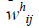of the hidden layer. In the neurons of the hidden layer, first the weighted sum of the inputs is computed: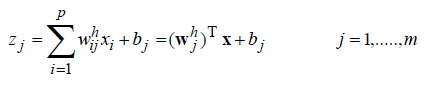(1)

It is then passed through a nonlinear activation function, such as the tangent hyperbolic: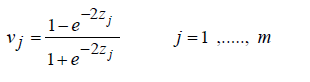(2)

Other typical activation functions are the threshold function (hard limiter) and the sigmoidal function. The neurons in the output layer are linear, i.e. only compute the weighted sum of their inputs: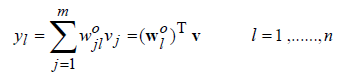(3)

You can train an ANN to perform a particular function by adjusting the values of the connections (weights) between elements, so that a particular input leads to a specific target output. The tuning of the ANN parameters (weight and bias) is called the training of the network. The most widely used training algorithm is the back-propagation algorithm. In this algorithm, data are processed through the network, until it reaches the output layer (forward pass). The resultant value of the output layer is compared to the target and the error is processed back through the network (backward pass) updating the individual weights of the connections and the biases of the individual neurons.

Fuzzy inference  is a process of mapping from a given input to an output using the fuzzy set methods. The fuzzification component transforms each crisp input variable into a membership grade based on the membership functions defined. The inference engine then conducts the fuzzy reasoning process by applying the appropriate fuzzy operators in order to obtain the fuzzy set to be accumulated in the output variable. The defuzzifier transforms the fuzzy output into a crisp output by applying a specific defuzzification method.

An ANFIS system can be considered as an implementation of a TS system in neural network architecture. In the following, we sketch briefly the outline of an ANFIS system using a model with two inputs as an example [24, 25]. Let the inputs of the fuzzy system be x and y, and let the output be f. We consider a TS system with first-order consequents as follows: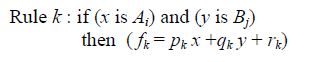(4)

Here, Ai and Bj are fuzzy sets describing the input and fk is a crisp (non-fuzzy) variable describing the output. In this structure, therefore, the inputs to the system are fuzzy, whereas the output is a crisp number. To construct the adaptive system, five layers are used as shown in Figure 3 for an illustrative ANFIS system using a model of two inputs and two rules. In this illustrative example, the number of if-then rules and the number of membership functions associated to each input were restricted to the number of the inputs (i.e k=i=j= 1,2). Each layer involves several nodes described by a node function. The circles in the network represent nodes that possess no variable parameters, while the squares represent nodes that possess adaptive parameters to be determined by the network during training. The node function in each layer is described below. Throughout the presentation, Oln denotes the output of node n in layer l.

Layer 1:

The nodes in this layer represent the fuzzy sets in the antecedents of the fuzzy rules. It has parameters that control the shape and the location of the centre of each fuzzy set. All the nodes in this layer are adaptive nodes. The output of each node n is the degree of membership of the input to the fuzzy membership function (MF) represented by the node: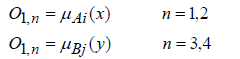(5)

where μAi(x) is the degree of membership of a variable x into the fuzzy set Ai. In this study, we choose μAi(x) to be Gaussian with height equal to 1. The membership function is given by: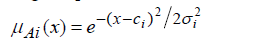(6)

where ci represents the centre of the Gaussian function, and σi represents the spread of the membership function.

Layer 2:

The nodes in this layer are fixed (not adaptive). They are labeled M to indicate that they play the role of a simple multiplier. The outputs of these nodes are given by: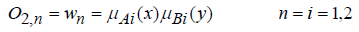(7)

The aim of this layer is to compute the degrees of activation (firing strength) of particular fuzzy rule.

Layer 3:

Nodes in this layer are also fixed nodes. They are labeled N to indicate that they perform a normalization of the firing strength of the rules, by calculating the ratio of the kth rule’s firing strength to the sum of all rules firing strengths. The output of each node in this layer is given by: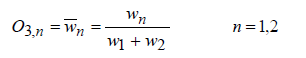(8)

Layer 4:

All the nodes in this layer are adaptive nodes. The output of each node in this layer is simply the product of the normalized firing strength and the crisp output: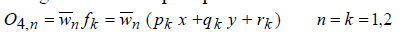(9)

where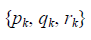is the parameter set.

Layer 5:

This layer has only one node, labeled S, to indicate that it performs the function of a simple summer. The output of this single node computes the overall output as the summation of all the inputs from the previous layer, i.e.,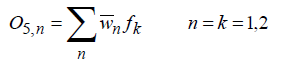(10)

In this study, a simple ANFIS Sugeno-type architecture has been implemented. Particularly, sixteen fuzzy ‘‘if-then’’ rules have been chosen, that govern the operation of processing a set of applied input variables, to produce a single predicted output which can then be compared to the corresponding measured (target or desired) output for validation purposes. The number of inputs to the ANFIS is two; represent the cable spacing and the cable burial depth. Each input variable attracts four parameterized Gaussian-shaped membership functions (MFs) that represent its fuzzy linguistic labels or values (i.e. low, medium-low, medium-high and high). The rule base used in the ANFIS model of this study is of the form that appears in equation (4). x and y are the inputs (cable spacing and burial depth), fk is the kth output of the kth fuzzy rule (k = 1,….,16). Ai and Bj (i=1,….,4 & i=1,…., 4) are the membership functions assigned to the two inputs, respectively. pk, qk, and rk (k= 1,….,16) constitute the set of linear parameters (consequent parameters). The premise parameters set consists of the collective parameters of all inputs’ Gaussian MF’s. Each MF possesses and is characterized by two nonlinear parameters (premise parameters) of the form which is defined in the equation (6) for Ai.

The overall predicted output-in this particular case can be extracted from equation (10) by extending the number of if-then rule to 16 as shown in equation (11)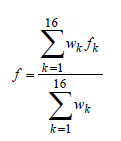(11)

where wk is the firing strength of the kth rule obtained by multiplying all membership grades of all inputs in that rule. Both the premise (nonlinear) and consequent (linear) parameters of the ANFIS should be tuned, utilizing the so-called learning process, to optimally represent the factual mathematical relationship between the input space and output space. Normally, as a first step, an approximate fuzzy model is initiated by the system and then improved through an iterative adaptive learning process. Basically, ANFIS takes the initial fuzzy model and tunes it by means of a hybrid technique combining gradient descent backpropagation and mean least squares optimization algorithms . At each epoch, an error measure, usually defined as the sum of the squared difference between actual and desired output, is reduced. Training stops when either the predefined epoch number or error rate is obtained.

Practical Application

Cable System Configuration

Two practical cable systems are used in this study. Figure 4 shows a schematic diagram of the cable geometry of the first cable system configuration.

Three cables of 13.8 kV, three phase, single core are directly buried in a trench and covered by backfill layer, which are surrounded by the native soil. The nominal values for the thermal resistivity are 1.7 and 1.3o C.m/W for the native soil and backfill respectively. S represents the direct distance between cable centers and L represents the cable burial depth. Figure 5 shows 15 kV, 3×300 mm2 CU/XLPE three phase, three cores cable system. The three cables are directly buried in the soil with the shown actual practical configuration. The mother soil, the upper backfill and the lower backfill thermal resistivities are 2, 0.95 and 0.8o C. m /W respectively.

Effect of Geometrical Parameter Variations

The effect of variations in the cable spacing and burial depth on the cable temperature of the cable system of Figure 4 is investigated. The spacing between cables has been changed from the allowable minimum value of 1 mm to the maximum feasible value of 0.2567 m at various values of the cable burial depth. The effect of cable spacing on the cable temperature is shown in Figure 6. It can be noted that the spacing has a positive effect on reducing the cables temperature due to the mutual heating reduction. The impact is greater on the upper cable than the other two lower cables. This can be interpreted by recalling that the increase in the cable spacing, in this triangular configuration case, will result in increase of the lower cable buried depth, whereas, it is the opposite for the upper cable, the buried depth will decrease and allow more heat convection. Furthermore, the influence of cable burial depth is of interest, the burial depth has been changed in the range between 1 mm and 1m at various values of the cable spacing as shown in Figure 7. It can be noted that increasing of buried depth has a negative effect on rising the cables temperature due to the overall increase of the thermal resistance between the cable surface and the ground interface.

Intelligent model results

The underlying model implemented in this study is completely based generated data using finite element method for different configurations in order to train and validate the two developed models (ANN and ANFIS). As described, the objective is to map a set of inputs (cable spacing and cable burial depth) to their corresponding outputs with the cable temperature being the target output of this prediction process. First, the developed AI technique has been implemented on the first cable system shown in Figure 4. In the case of BPNN, there is an input layer with two neurons, representing the two inputs, an output layer, with one neuron representing the cable temperature. One hidden layers as intermediate layers are also included. For both cable systems under investigation, the trials showed that the one-hidden layer network performs significantly better than the multi-hidden layers network. The network with twenty, nodes in the hidden layer gave the optimal configuration with the minimum mean square error (MSE) for first cable systems considered in figure 4. Figure 8 shows the structure of the proposed BPNN model. The back-propagation neural network is trained by feeding a set of mapping data with input and target variables. The main objective of training the neural network is to assign the connection weights by reducing the errors between the predicted and actual target values to a satisfactory level. This process is carried out through the minimization of the defined error function by updating the connection weights. Also, the number of hidden layers, number of hidden nodes, transfer functions, and normalization of data are chosen to get the best performance of the model. The network has been trained continually through the updating weights. On the other hand, in the development of the ANFIS model, Four Gaussian-shaped membership functions for each input variable were selected. Since the system has two inputs with four membership functions for each input, there will be a total sixteen fuzzy ‘‘if-then’’ rules represent all possible combinations. Figure 9 presents architecture of the adaptive neuro-fuzzy inference system to the cable temperature. Figure 10 depicts the initial membership functions of the burial depth input which are equally spaced along the normalized range of the prospective input variable.

Both ANN and AFIS Network architectures were optimized in terms of the design parameters based on iterative approach. In order to show the applicability of the two developed AI techniques, they were implemented on the cable system of Figure 5. The optimal network structure for the ANN is one hidden layer with six neurons, whereas two MF was used for the ANFIS simulation.

In order to validate the ANN and ANFIS models, the two models are tested with a separate set of testing data that is not used in the training phase. The deviation of the cable temperature of ANN and ANFIS from that calculated using the FE is carried out at various values of the cable spacing and burial depth. Figure 11 and Figure 12 show comparison of the ANN deviation against ANFIS deviation both from the FE solution for both cable systems. Table .1 shows the mean square error and the maximum absolute error of ANN and ANFIS of the two cable systems.

In order to show the effectiveness of the two introduced intelligent techniques in terms of the computational time, a comparison with finite element has been conducted. In this first case study, a finite element mesh of 9423 nodes and 18746 elements were utilized for the domain discretization, and consequently a liner system of equations with 9423 unknowns, represent the node temperatures is needed to be solved 256 times according to the input set size which consist of various combination of the input cable spacing and buried depth.

The computation times for are 33.1 and 7.7 seconds for the ANN and ANFIS respectively, whereas 2.1607×104 sec is needed in case of using the FE. In the case of the cable system# 2, the input set size is 638, the size of the mesh is 14,265 nodes and the finite element simulation time is 155148 sec. On the other hand, the simulation time of the ANN and ANFIS are 320.4 and 6.1 seconds respectively. Obviously, the FE method under parameter variations will be costly in terms of computational efforts as the number of inputs increases. On the other hand, the intelligent techniques can be adopted as effective alternative to the FE as it provides correspondence results with much less commotional complexity and execution time.

Discussion and Findings

In this paper, ANFIS and ANN are addressed in order to estimate the thermal field of underground power cable. The proposed AI methods were also compared with a conventional FE. Results have shown high correspondence with the conventional FE. The model has been applied on two different practical cable systems in terms of cable specifications, structure and system configuration.

In the case of thermal study of underground power cable, most of the thermal parameters are subjected to variations from time to time, such as the ambient temperature and the moisture contents. Furthermore, variations encounter as a sequence of natural difference of the soil and due to expected dislocation of the cable and consequently, changing in cable depth and spacing along the cable route. Finite elements method can deal with varying properties including the complexity of the system. However, in case of variability of the parameters, the finite element is timely consuming and expensive in terms of the computations. The major advantage of AI is their ability to interpret data and facilitate corrective action without resorting to a mathematical model.

AI techniques can be used in conjunction with limited FE analysis to capture the effect of influential parameter variations and provide a solution for such complex mapping avoiding the extensive numerical computations.

In the proposed algorithm, FE calculations, has not been limited to cable temperature, in fact, it covers geometrically area around the cable including mother soil. On the other hand, AI is limited to capture the trend of the cable temperature due to variation of selected input parameters. Furthermore, FE needs full profile of the model characteristic (geometrical, boundary and medium specifications) which have to be provided as inputs. For AI, the important parameters that have the great influence on the cable performance will only be utilized to generalize the problem with the minimum structure complexity. The input parameters are subjected to distribution in the whole solution area of the above problem, only the necessary fuzzy rules- in case of ANFISwithin the premise space is included, leading to a minimum of training parameters. It is worth mentioning that input space partitioning for both ANFIS and ANN and the size of rule-base for ANFIS are crucial for computational expense. Consequently, they are appropriate for problems having relatively small number of input variables and/or involving small to medium number of training patterns.

In conclusion, FE is superior in terms of modeling precision, whereas the AI comes in the picture due to its capability in terms of uncertainty handling, computational expense, and data requirements. Results indicated that, both techniques are valid and have certain advantages over each other and should be preferred with respect to quantity and quality of the data at hand. This paper introduces merging of numerical and artificial intelligence techniques in one comprehensive module in order to gain the unique advantages of both. The developed method can be a very efficient tool for the study of thermal fields under various underground power cable system configurations and useful alternative in circumstances of model parameter variations. Further Advantage of this method would be obvious when combine with underground cable online monitoring when measurement data set for network training is available. The proposed intelligence system might also be adapted to similar studies for analysis and design of electric power systems.

#### Conclusion

This paper has presented a comparative evaluation of two intelligent techniques, which are newly introduced in this paper for thermal assessment of power cables. The two techniques employ the back-propagation neural network (BPNN) and adaptive neuro-fuzzy inference system (ANFIS) models, which are developed to predict the cable temperature under geometrical parameter variations. The effect of cable spacing and cable burial depth on cable ampacity has been investigated. The two models are trained using an input-output pattern generated using FE method, which is extensively used in this field of study. In the work of the paper, a portion of the FE-generated output results, which have not been provided to the models – as input data - in the training phase, were utilized to compare the cable temperature of the three methods (FE, ANN and ANFIS). The results presented in the paper for the two intelligent methods have shown high correspondence with the finite element solution. This conclusion confirms that the introduction of the intelligent techniques provides a reliable and simple alternative approach for the thermal field evaluation by avoiding numerous computational complexities of the numerical methods.

#### References

Select your language of interest to view the total content in your interested language

### Article Usage

• Total views: 12294
• [From(publication date):
December-2012 - Dec 06, 2019]
• Breakdown by view type
• HTML page views : 8483Can't read the image? click here to refresh